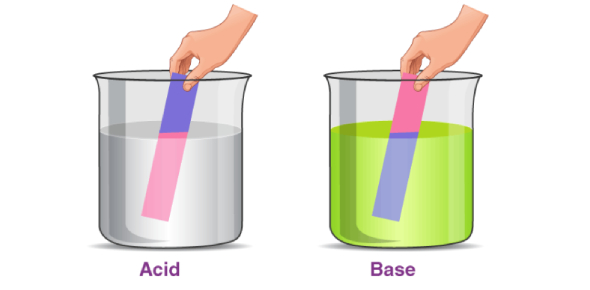# Acids & Bases Quick Quiz

14 Questions | Total Attempts: 1895SettingsWhat are acids and bases? What are some properties of acids and bases?

• 1.
Which statement is true of all strong acids in solution?
• A.

Carbon dioxide is given off when strong acids react with carbonates.

• B.

Ammonia is given off when they react with ammonium salts.

• C.

They produce hydrogen on reaction with any metal.

• D.

They have a pH greater than 7

• 2.
A sample of air was bubbled into pure water. The pH of the water slowly changed from 7 to 6. Which gas in the sample caused this change?
• A.

Argon

• B.

Carbon dioxide

• C.

Nitrogen

• D.

Carbon Monoxide

• 3.
Dilute sulphuric acid reacts with copper (II) oxide and with copper (II) carbonate. In what way are these two reactions alike?
• A.

An acid is neutralised by an alkali.

• B.

A gas is produced.

• C.

Water is produced.

• 4.
Which of the following compounds dissolves in water to give a solution with pH greater than 7?
• A.

Calcium carbonate

• B.

Copper (II) hydroxide

• C.

Sodium hydroxide

• D.

Silver chloride

• 5.
Four aqueous solutions have the pH values shown below. If pairs of the solutions are mixed, which pair must produce an acidic mixture?Solution P    Solution Q    Solution R    Solution SpH:      2               6                  8                 10
• A.

P and Q

• B.

P and R

• C.

P and S

• D.

Q and R

• 6.
Under suitable conditions, hydrochloric acid reacts with each of the substances. Which reaction gives a colourless solution only?
• A.

Calcium carbonate

• B.

Copper (II) oxide

• C.

Potassium hydroxide

• 7.
Which of the following substances is used to decrease the acidity in a soil?
• A.

Ammonium nitrate

• B.

Magnesium chloride

• C.

Calcium hydroxide

• D.

Potassium chloride

• 8.
Which of the following is a characteristic property of alkalis in aqueous solution?
• A.

They liberate (give off) ammonia when reacted with ammonium salts.

• B.

They liberate carbon dioxide when reacted with carbonates.

• C.

They produce hydrogen with any metal.

• D.

They turn Universal Indicator paper red.

• 9.
The pH of a solution of hydrochloric acid is 2. What will be the pH of the acid after 10g of sodium chloride is added to it?
• A.

1

• B.

2

• C.

7

• D.

9

• 10.
Give the formula of the ion which is produced in aqueous solution (when dissolved in water) byan alkali.
• 11.
State the products for the reaction between magnesium carbonate and hydrochloric acid.
• 12.
Alkalis are water-soluble ______.
• 13.
______ is the chemical reaction between hydrogen and hydroxide ions.
• 14.
Adding hydrochloric acid to sodium hydroxide _____ the pH value.
Related TopicsBack to top